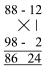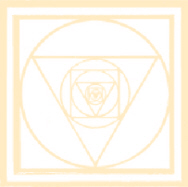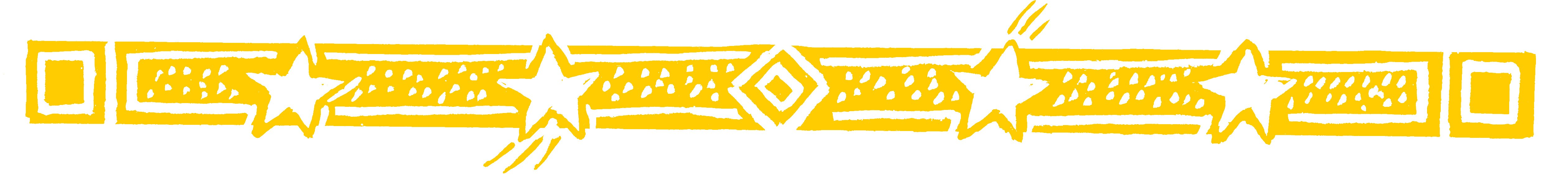AT A PARTYAt a party surprise your friends with this spectacular way of multiplying large numbers together in your head.

• Suppose you want to multiply 88 by 98.

Not easy,you might think. But with
VERTICALLY AND CROSSWISE you can give
the answer immediately, using the same method
as before.

Both 88 and 98 are close to 100.
88 is 12 below 100 and 98 is 2 below 100.

You can imagine the sum set out like this:As before the 86 comes from
subtracting crosswise: 88 - 2 = 86
(or 98 - 12 = 86: you can subtract
either way, you will always get
And the 24 in the answer is
just 12 x 2: you multiply vertically.
So 88 x 98 = 8624This is so easy it is just mental arithmetic.

Question Comment !
 Tutorial Title Question Title No Questions 0 Questions Asked 0 Skipped Questions 0 Correctly Answered 0 Wrongly Answered 0 Total Question Attempts 0 Current Question Attempts 0

Skip Question

Reset Test

Halt Test

 No Questions 0 Questions Asked 0 Skipped Questions 0 Correctly Answered 0 Wrongly Answered 0 Total Question Attempts 0

Time Taken

CloseAt A PartyWhere there is life there is pattern
and where there is pattern there is
mathematics
John D. Barrow